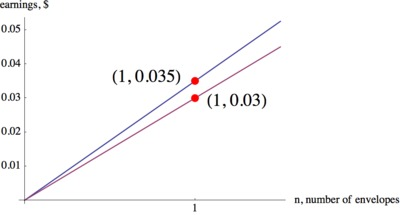# Stuffing Envelopes

Alignments to Content Standards: 8.EE.B.5 8.EE.B

Anna and Jason have summer jobs stuffing envelopes for two different companies. Anna earns \$14 for every 400 envelops she finishes. Jason earns \$9 for every 300 envelopes he finishes.
1. Draw graphs and write equations that show the earnings, $y$ as functions of the number of envelopes stuffed, $n$ for Anna and Jason.
3. Suppose Anna has savings of \$100 at the beginning of the summer and she saves all her earnings from her job. Graph her savings as a function of the number of envelopes she stuffed,$n$. How does this graph compare to her previous earnings graph? What is the meaning of the slope in each case? ## IM Commentary Students learn about proportional relationships and explore them through tables, graphs, and equations in 6th and 7th grade. A proportional relationship can be thought of as a linear relationship whose graph goes through the origin. Students make the step from proportional relationships in particular to linear functions in general in 8th grade. As part of this transition, students should recognize the slope of a line through the origin as the unit rate for that proportional relationship. From there they learn that the slope of any line can be interpreted as the rate of change of the corresponding linear relationship. This task provides students with an opportunity to take the step from unit rates in a proportional relationship to the rate of change of a linear relationship. Students should already be familiar with proportional relationships from their work in prior grades. In part (b) they are asked to examine the graphs more closely and verbalize how they are different, and how this difference reflects the situation (this is work they have already done; see 7.RP.2). If the teacher wishes to use this task to introduce slope, it would be appropriate to formally define the concept of slope after students have worked on part (b) of the task. The teacher should note that the students are already familiar with the connection between the slope and the constant of proportionality in a proportional relationship. Next, the teacher can extend the idea of slope to any line defined by an equation of the form$y=mx+b$. (Note that showing that the slope of a line is well-defined requires a geometric argument that is not addressed by this task; see 8.EE.6.) This is accomplished in the task by extending the example of Anna's summer earnings to her summer savings. It is easy to see that the line that represents her savings as a function of the number of envelopes she stuffs will have the same slope as the previous earnings line; the difference will be in the interpretation of the slope. For her earnings, she will make 3.5 cents for every envelope she stuffs, but for her savings, she will save an additional 3.5 cents for every additional envelope she stuffs. This subtle difference in the interpretation of the slope signals the difference between a proportional relationship and a relationship that is not proportional but changes proportionally. ## Solution 1. The amount of money earned,$y,$and the number of envelopes stuffed,$n,$are proportional to each other. Since Anna earns \$14 for 400 envelope, she makes $\frac{14}{400} = 0.035$ dollars per envelope. Therefore, we have $y=0.035n$ for Anna's equation.
Jason earns \$9 for every 300 envelopes he stuffs, so he makes$\frac{9}{300}= 0.03$dollars per envelope. So we have$y=0.03n$for Jason's equation. Since Anna's equation has a larger unit rate, 0.035 dollars per envelope vs. 0.03 dollars per envelope for Jason, she has the higher paying job. The graphs of the equations are shown below.2. We know that we can find the unit rate of proportional relationships by finding the point on the line with horizontal coordinate 1, as shown in the graph below.For every envelope they stuff, Anna makes half a cent more than Jason. Since Anna makes more money per envelope, her earnings increase faster than Jason's. Therefore, her earnings line is steeper than Jason's. 3. Anna still earns money at the same rate as before, but now her earnings are added to her savings of \$100. The graph showing her total savings, including the money she earns, is still linear but it has a higher starting value. The new line is parallel to the previous earnings line but while the previous line went through the point $(0,0)$, the new line starts at the point $(0, 100)$. This shows that when she starts working she already has \$100 in savings.For her earnings graph, we see that she will make \$0.035 for every envelope she stuffs, but for her savings, she will save an additional \\$0.035 for every additional envelope she stuffs.